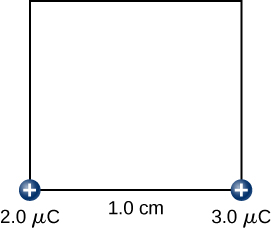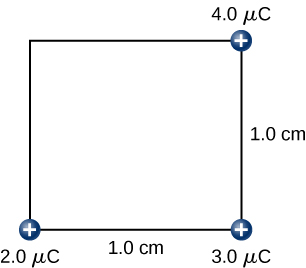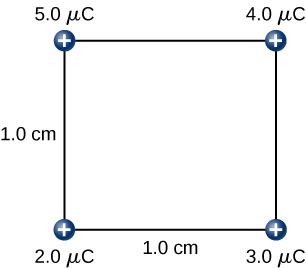# 7.1 Electric potential energy  (Page 4/5)

 Page 4 / 5
${W}_{2}=k\frac{{q}_{1}{q}_{2}}{{r}_{12}}=\left(9.0\phantom{\rule{0.2em}{0ex}}×\phantom{\rule{0.2em}{0ex}}{10}^{9}\frac{\text{N}·{\text{m}}^{2}}{{\text{C}}^{2}}\right)\frac{\left(2.0\phantom{\rule{0.2em}{0ex}}×\phantom{\rule{0.2em}{0ex}}{10}^{-6}\phantom{\rule{0.2em}{0ex}}\text{C}\right)\left(3.0\phantom{\rule{0.2em}{0ex}}×\phantom{\rule{0.2em}{0ex}}{10}^{-6}\phantom{\rule{0.2em}{0ex}}\text{C}\right)}{1.0\phantom{\rule{0.2em}{0ex}}×\phantom{\rule{0.2em}{0ex}}{10}^{-2}\phantom{\rule{0.2em}{0ex}}\text{m}}=5.4\phantom{\rule{0.2em}{0ex}}\text{J}\text{.}$Step 2. Work W 2 to bring the + 3.0 - μ C charge from infinity.

Step 3. While keeping the charges of $+2.0\phantom{\rule{0.2em}{0ex}}\mu \text{C}$ and $+3.0\phantom{\rule{0.2em}{0ex}}\mu \text{C}$ fixed in their places, bring in the $+4.0\text{-}\mu \text{C}$ charge to $\left(x,y,z\right)=\left(1.0\phantom{\rule{0.2em}{0ex}}\text{cm},1.0\phantom{\rule{0.2em}{0ex}}\text{cm},0\right)$ ( [link] ). The work done in this step is

$\begin{array}{cc}{W}_{3}\hfill & =k\frac{{q}_{1}{q}_{3}}{{r}_{13}}+k\frac{{q}_{2}{q}_{3}}{{r}_{23}}\hfill \\ & =\left(9.0\phantom{\rule{0.2em}{0ex}}×\phantom{\rule{0.2em}{0ex}}{10}^{9}\frac{\text{N}·{\text{m}}^{2}}{{\text{C}}^{2}}\right)\left[\frac{\left(2.0\phantom{\rule{0.2em}{0ex}}×\phantom{\rule{0.2em}{0ex}}{10}^{-6}\phantom{\rule{0.2em}{0ex}}\text{C}\right)\left(4.0\phantom{\rule{0.2em}{0ex}}×\phantom{\rule{0.2em}{0ex}}{10}^{-6}\phantom{\rule{0.2em}{0ex}}\text{C}\right)}{\sqrt{2}\phantom{\rule{0.2em}{0ex}}×\phantom{\rule{0.2em}{0ex}}{10}^{-2}\phantom{\rule{0.2em}{0ex}}\text{m}}+\frac{\left(3.0\phantom{\rule{0.2em}{0ex}}×\phantom{\rule{0.2em}{0ex}}{10}^{-6}\phantom{\rule{0.2em}{0ex}}\text{C}\right)\left(4.0\phantom{\rule{0.2em}{0ex}}×\phantom{\rule{0.2em}{0ex}}{10}^{-6}\phantom{\rule{0.2em}{0ex}}\text{C}\right)}{1.0\phantom{\rule{0.2em}{0ex}}×\phantom{\rule{0.2em}{0ex}}{10}^{-2}\phantom{\rule{0.2em}{0ex}}\text{m}}\right]=15.9\phantom{\rule{0.2em}{0ex}}\text{J}\text{.}\hfill \end{array}$Step 3. The work W 3 to bring the + 4.0 - μ C charge from infinity.

Step 4. Finally, while keeping the first three charges in their places, bring the $+5.0\text{-}\mu \text{C}$ charge to $\left(x,y,z\right)=\left(0,\phantom{\rule{0.2em}{0ex}}1.0\phantom{\rule{0.2em}{0ex}}\text{cm},\phantom{\rule{0.2em}{0ex}}0\right)$ ( [link] ). The work done here is

$\begin{array}{cc}{W}_{4}\hfill & =k{q}_{4}\left[\frac{{q}_{1}}{{r}_{14}}+\frac{{q}_{2}}{{r}_{24}}+\frac{{q}_{3}}{{r}_{34}}\right],\hfill \\ & =\left(9.0\phantom{\rule{0.2em}{0ex}}×\phantom{\rule{0.2em}{0ex}}{10}^{9}\frac{\text{N}·{\text{m}}^{2}}{{\text{C}}^{2}}\right)\left(5.0\phantom{\rule{0.2em}{0ex}}×\phantom{\rule{0.2em}{0ex}}{10}^{-6}\phantom{\rule{0.2em}{0ex}}\text{C}\right)\left[\frac{\left(2.0\phantom{\rule{0.2em}{0ex}}×\phantom{\rule{0.2em}{0ex}}{10}^{-6}\phantom{\rule{0.2em}{0ex}}\text{C}\right)}{1.0\phantom{\rule{0.2em}{0ex}}×\phantom{\rule{0.2em}{0ex}}{10}^{-2}\phantom{\rule{0.2em}{0ex}}\text{m}}+\frac{\left(3.0\phantom{\rule{0.2em}{0ex}}×\phantom{\rule{0.2em}{0ex}}{10}^{-6}\phantom{\rule{0.2em}{0ex}}\text{C}\right)}{\sqrt{2}\phantom{\rule{0.2em}{0ex}}×\phantom{\rule{0.2em}{0ex}}{10}^{-2}\phantom{\rule{0.2em}{0ex}}\text{m}}+\frac{\left(4.0\phantom{\rule{0.2em}{0ex}}×\phantom{\rule{0.2em}{0ex}}{10}^{-6}\phantom{\rule{0.2em}{0ex}}\text{C}\right)}{1.0\phantom{\rule{0.2em}{0ex}}×\phantom{\rule{0.2em}{0ex}}{10}^{-2}\phantom{\rule{0.2em}{0ex}}\text{m}}\right]=36.5\phantom{\rule{0.2em}{0ex}}\text{J}\text{.}\hfill \end{array}$Step 4. The work W 4 to bring the + 5.0 - μ C charge from infinity.

Hence, the total work done by the applied force in assembling the four charges is equal to the sum of the work in bringing each charge from infinity to its final position:

${W}_{\text{T}}={W}_{1}+{W}_{2}+{W}_{3}+{W}_{4}=0+5.4\phantom{\rule{0.2em}{0ex}}\text{J}+15.9\phantom{\rule{0.2em}{0ex}}\text{J}+36.5\phantom{\rule{0.2em}{0ex}}\text{J}=57.8\phantom{\rule{0.2em}{0ex}}\text{J}\text{.}$

## Significance

The work on each charge depends only on its pairwise interactions with the other charges. No more complicated interactions need to be considered; the work on the third charge only depends on its interaction with the first and second charges, the interaction between the first and second charge does not affect the third.

Check Your Understanding Is the electrical potential energy of two point charges positive or negative if the charges are of the same sign? Opposite signs? How does this relate to the work necessary to bring the charges into proximity from infinity?

positive, negative, and these quantities are the same as the work you would need to do to bring the charges in from infinity

Note that the electrical potential energy is positive if the two charges are of the same type, either positive or negative, and negative if the two charges are of opposite types. This makes sense if you think of the change in the potential energy $\text{Δ}U$ as you bring the two charges closer or move them farther apart. Depending on the relative types of charges, you may have to work on the system or the system would do work on you, that is, your work is either positive or negative. If you have to do positive work on the system (actually push the charges closer), then the energy of the system should increase. If you bring two positive charges or two negative charges closer, you have to do positive work on the system, which raises their potential energy. Since potential energy is proportional to 1/ r , the potential energy goes up when r goes down between two positive or two negative charges.

On the other hand, if you bring a positive and a negative charge nearer, you have to do negative work on the system (the charges are pulling you), which means that you take energy away from the system. This reduces the potential energy. Since potential energy is negative in the case of a positive and a negative charge pair, the increase in 1/ r makes the potential energy more negative, which is the same as a reduction in potential energy.

The result from [link] may be extended to systems with any arbitrary number of charges. In this case, it is most convenient to write the formula as

${W}_{12\cdots N}=\frac{k}{2}\sum _{i}^{N}\sum _{j}^{N}\frac{{q}_{i}{q}_{j}}{{r}_{ij}}\phantom{\rule{0.2em}{0ex}}\text{for}\phantom{\rule{0.2em}{0ex}}i\ne j.$

The factor of 1/2 accounts for adding each pair of charges twice.

## Summary

• The work done to move a charge from point A to B in an electric field is path independent, and the work around a closed path is zero. Therefore, the electric field and electric force are conservative.
• We can define an electric potential energy, which between point charges is $U\left(r\right)=k\frac{qQ}{r}$ , with the zero reference taken to be at infinity.
• The superposition principle holds for electric potential energy; the potential energy of a system of multiple charges is the sum of the potential energies of the individual pairs.

## Conceptual questions

Would electric potential energy be meaningful if the electric field were not conservative?

No. We can only define potential energies for conservative fields.

Why do we need to be careful about work done on the system versus work done by the system in calculations?

Does the order in which we assemble a system of point charges affect the total work done?

No, though certain orderings may be simpler to compute.

## Problems

Consider a charge ${Q}_{1}\left(+5.0\phantom{\rule{0.2em}{0ex}}\mu \text{C}\right)$ fixed at a site with another charge ${Q}_{2}$ (charge $+3.0\phantom{\rule{0.2em}{0ex}}\mu \text{C}$ , mass $6.0\phantom{\rule{0.2em}{0ex}}\mu \text{g}\right)$ moving in the neighboring space. (a) Evaluate the potential energy of ${Q}_{2}$ when it is 4.0 cm from ${Q}_{1}.$ (b) If ${Q}_{2}$ starts from rest from a point 4.0 cm from ${Q}_{1},$ what will be its speed when it is 8.0 cm from ${Q}_{1}$ ? ( Note: ${Q}_{1}$ is held fixed in its place.)

a. $U=3.4\phantom{\rule{0.2em}{0ex}}\text{J;}$
b. $\frac{1}{2}m{v}^{2}=k{Q}_{1}{Q}_{2}\left(\frac{1}{{r}_{i}}-\frac{1}{{r}_{f}}\right)\to v=750\phantom{\rule{0.2em}{0ex}}\text{m/s}$

Two charges ${Q}_{1}\left(+2.00\phantom{\rule{0.2em}{0ex}}\mu \text{C}\right)$ and ${Q}_{2}\left(+2.00\phantom{\rule{0.2em}{0ex}}\mu \text{C}\right)$ are placed symmetrically along the x -axis at $x=±3.00\phantom{\rule{0.2em}{0ex}}\text{cm}$ . Consider a charge ${Q}_{3}$ of charge $+4.00\phantom{\rule{0.2em}{0ex}}\mu \text{C}$ and mass 10.0 mg moving along the y -axis. If ${Q}_{3}$ starts from rest at $y=2.00\phantom{\rule{0.2em}{0ex}}\text{cm,}$ what is its speed when it reaches $y=4.00\phantom{\rule{0.2em}{0ex}}\text{cm?}$

To form a hydrogen atom, a proton is fixed at a point and an electron is brought from far away to a distance of $0.529\phantom{\rule{0.2em}{0ex}}×\phantom{\rule{0.2em}{0ex}}{10}^{-10}\phantom{\rule{0.2em}{0ex}}\text{m,}$ the average distance between proton and electron in a hydrogen atom. How much work is done?

$U=4.36\phantom{\rule{0.2em}{0ex}}×\phantom{\rule{0.2em}{0ex}}{10}^{-18}\phantom{\rule{0.2em}{0ex}}\text{J}\phantom{\rule{0.2em}{0ex}}$

(a) What is the average power output of a heart defibrillator that dissipates 400 J of energy in 10.0 ms? (b) Considering the high-power output, why doesn’t the defibrillator produce serious burns?

Maxwell's stress tensor is
if 6.0×10^13 electrons are placed on a metal sphere of charge 9.0micro Coulombs, what is the net charge on the sphere
18.51micro Coulombs
ASHOK
Is it possible to find the magnetic field of a circular loop at the centre by using ampere's law?
Is it possible to find the magnetic field of a circular loop at it's centre?
yes
Brother
The density of a gas of relative molecular mass 28 at a certain temperature is 0.90 K kgmcube.The root mean square speed of the gas molecules at that temperature is 602ms.Assuming that the rate of diffusion of a gas in inversely proportional to the square root of its density,calculate the density of
A hot liquid at 80degree Celsius is added to 600g of the same liquid originally at 10 degree Celsius. when the mixture reaches 30 degree Celsius, what will be the total mass of the liquid?
what is electrostatics
Study of charges which are at rest
himanshu
Explain Kinematics
Two equal positive charges are repelling each other. The force on the charge on the left is 3.0 Newtons. Using your notes on Coulomb's law, and the forces acting on each of the charges, what is the force on the charge on the right?
Using the same two positive charges, the left positive charge is increased so that its charge is 4 times LARGER than the charge on the right. Using your notes on Coulomb's law and changes to the charge, once the charge is increased, what is the new force of repulsion between the two positive charges?
Nya
A mass 'm' is attached to a spring oscillates every 5 second. If the mass is increased by a 5 kg, the period increases by 3 second. Find its initial mass 'm'
a hot water tank containing 50,000g of water is heated by an electric immersion heater rated at 3kilowatt,240volt, calculate the current
what is charge
product of current and time
Jaffar
Why always amber gain electrons and fur loose electrons? Why the opposite doesn't happen?
A closely wound search coil has an area of 4cm^2,1000 turns and a resistance of 40ohm. It is connected to a ballistic galvanometer whose resistance is 24 ohm. When coil is rotated from a position parallel to uniform magnetic field to one perpendicular to field,the galvanometer indicates a charge
Using Kirchhoff's rules, when choosing your loops, can you choose a loop that doesn't have a voltage?
how was the check your understand 12.7 solved?By Donyea SweetsBy OpenStaxBy Richley CrapoBy Janet ForresterBy Brooke DelaneyBy OpenStaxBy Maureen MillerBy Janet ForresterBy Tess ArmstrongBy OpenStax